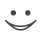# Get these flashcards, study & pass exams. For free! Even on iPhone/Android!

## Population Dynamics (8 Cards)

What is Z
The total instantaneous mortality rate.  Take the natural log of the number of survivors each age class and calculate the slope of the line.  Z=slope and is always negative.
What is stable age distribution?
When there is a constant proportion of each age class in the population, even if there is growth or decline in the total population.  Find by calculating the proportions for each year and comparing the proportions.  If proportions at time t are the same at time t+1, you have reached the stable age distribution.
How do you calculate the number of 1 year olds at t+1 for a pre-breeding census model?
The number of 1 year olds at time t+1 is equal to the number of adults at time t, times the fecundity, times the survival rate of newborns to age 1.
How is Z different from r?
Z measures the decrease in cohort or age class size over time while r measures the change in population size through time.
How are Z and r similar?
Z and r are found by fitting a line to the natural log transformed data of either cohorts or population.  They are instantaneous rates - the slope of the line.
What is a catch curve analysis?
Done by sampling a population and counting the number of individuals in each age class.  Transform the data and fit a line to it.  The slope of the line gives you Z.
What are the assumptions of a catch curve analysis?
The mortality rate is constant across all age classes.  The mortality rate is constant over time.  Population is at a stable lambda.
What is a cohort analysis?
A cohort analysis requires several years of data.  You follow what cohort through time and plot the number of individuals in that cohort through time.  The slop of the ln of the cohort size is Z.Flashcard set info:
Author: fireflyally7
Main topic: Ecology
Topic: Population Dynamics
School / Univ.: Oregon State University
City: Corvallis
Published: 22.02.2010

Card tags:
All cards (8)
no tagsReport abuse